##### The Japanese Maple specialist
Direct order Contact Help / Services Newsletter# Shelf to displaySearch

Japanese MaplesAcer seeds and more Young maples and bonsaiHostasWind bells and chimesSaxifragesVarieties introduced into Europe

Packing and shipping charges

# 50 seeds of pinus densiflora

Pinus densiflora pin rouge du japon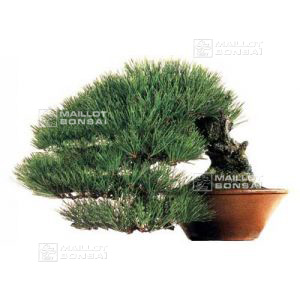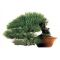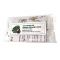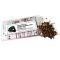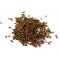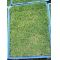ref. : 5291

8,00

Available quantity : 55Order

###### Description

Sachet of + - 50 very cool seeds of Japan of pinus densiflora. Commonly named aka matsu or red pine of Japan. Instructions for use include in the sachet. The seedling takes place in terrine after having placed the seeds in a plastic sachet with the cool sand in the ferry to vegetables of the refrigerator and this during 2 months. Then then to make the seedling in one soil draining to ambient temperature. Raised in 30/40 days. Recording in individual pot at the end of  first  year. To avoid the excesses of water.

#seeds 6.5 #densiflora 4 #sachet 3.6 #pinus 3.5 #seedling 2.8 #japan 2.5 #acer 2.4 #then 2.4 #more 2.4 #cool 2.4

Formule
(( ROUND((CHAR_LENGTH(b.article_nom)-CHAR_LENGTH(REPLACE(b.article_nom, 'seeds', '')))/LENGTH('seeds')) + ROUND((CHAR_LENGTH(b.article_description)-CHAR_LENGTH(REPLACE(b.article_description, 'seeds', '')))/LENGTH('seeds')) ) * 4.5) + (( ROUND((CHAR_LENGTH(b.article_nom)-CHAR_LENGTH(REPLACE(b.article_nom, 'densiflora', '')))/LENGTH('densiflora')) + ROUND((CHAR_LENGTH(b.article_description)-CHAR_LENGTH(REPLACE(b.article_description, 'densiflora', '')))/LENGTH('densiflora')) ) * 4) + (( ROUND((CHAR_LENGTH(b.article_nom)-CHAR_LENGTH(REPLACE(b.article_nom, 'sachet', '')))/LENGTH('sachet')) + ROUND((CHAR_LENGTH(b.article_description)-CHAR_LENGTH(REPLACE(b.article_description, 'sachet', '')))/LENGTH('sachet')) ) * 3.6) + (( ROUND((CHAR_LENGTH(b.article_nom)-CHAR_LENGTH(REPLACE(b.article_nom, 'pinus', '')))/LENGTH('pinus')) + ROUND((CHAR_LENGTH(b.article_description)-CHAR_LENGTH(REPLACE(b.article_description, 'pinus', '')))/LENGTH('pinus')) ) * 3.5) + (( ROUND((CHAR_LENGTH(b.article_nom)-CHAR_LENGTH(REPLACE(b.article_nom, 'seedling', '')))/LENGTH('seedling')) + ROUND((CHAR_LENGTH(b.article_description)-CHAR_LENGTH(REPLACE(b.article_description, 'seedling', '')))/LENGTH('seedling')) ) * 2.8) + (( ROUND((CHAR_LENGTH(b.article_nom)-CHAR_LENGTH(REPLACE(b.article_nom, 'japan', '')))/LENGTH('japan')) + ROUND((CHAR_LENGTH(b.article_description)-CHAR_LENGTH(REPLACE(b.article_description, 'japan', '')))/LENGTH('japan')) ) * 2.5) + (( ROUND((CHAR_LENGTH(b.article_nom)-CHAR_LENGTH(REPLACE(b.article_nom, 'cool', '')))/LENGTH('cool')) + ROUND((CHAR_LENGTH(b.article_description)-CHAR_LENGTH(REPLACE(b.article_description, 'cool', '')))/LENGTH('cool')) ) * 2.4) + (( ROUND((CHAR_LENGTH(b.article_nom)-CHAR_LENGTH(REPLACE(b.article_nom, 'then', '')))/LENGTH('then')) + ROUND((CHAR_LENGTH(b.article_description)-CHAR_LENGTH(REPLACE(b.article_description, 'then', '')))/LENGTH('then')) ) * 2.4) + (( ROUND((CHAR_LENGTH(b.article_nom)-CHAR_LENGTH(REPLACE(b.article_nom, 'instructions', '')))/LENGTH('instructions')) + ROUND((CHAR_LENGTH(b.article_description)-CHAR_LENGTH(REPLACE(b.article_description, 'instructions', '')))/LENGTH('instructions')) ) * 2.2) + (( ROUND((CHAR_LENGTH(b.article_nom)-CHAR_LENGTH(REPLACE(b.article_nom, 'refrigerator', '')))/LENGTH('refrigerator')) + ROUND((CHAR_LENGTH(b.article_description)-CHAR_LENGTH(REPLACE(b.article_description, 'refrigerator', '')))/LENGTH('refrigerator')) ) * 2.2)

## Secure payment## Delivery

Our logistic partners :